# Lions Weekly: June 2022# A Note From Mr. Sandefur

Lion Family,

Summertime is officially here, and I hope that each and every one of you is enjoying the beginning of what I pray is a beautiful summer. I am truly thankful for each and every one of our partners here at The King’s Academy and your faithfulness as we seek to develop servant leaders who will change the world for Christ. As we enter this time of preparation on campus, we are excited about several projects that are underway to improve the school experience for all stakeholders. Projects include but are not limited to Woody Auditorium facelift, the installation of a new electronic sign at the Boyd’s Creek entrance, multiple painting and repair projects, and a few other necessary maintenance projects.

I wanted to let you know that this will be the only newsletter sent this month; however special notices will be sent out periodically regarding new hires, etc. Our weekly newsletters will resume during the month of July as we prepare for the upcoming school year. In this newsletter you will find important dates and information to be aware of, so please take some time and familiarize yourself with the contents.

In Christ Alone,

Jeremy Sandefur
President

 table div table+table+table+table div table{width:100%;padding:0}table div table+table+table+table div table img{width:96.23%;padding:0;float:none}table div table+table+table+table div table td{width:100%;padding:0 1.88% 18px}/* styles */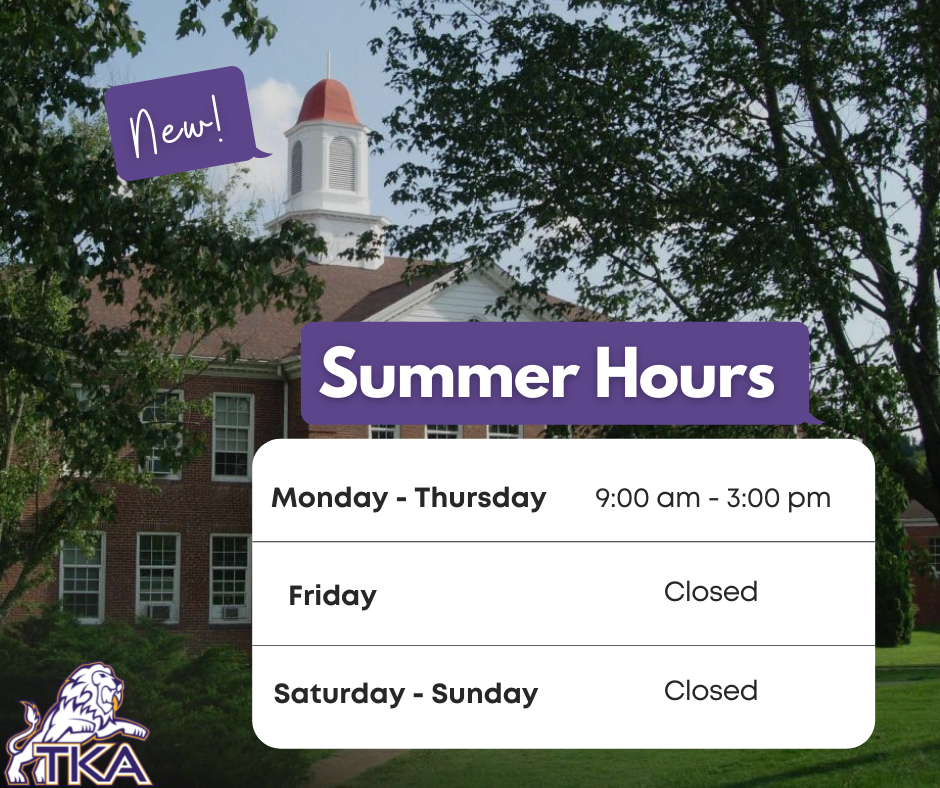# Summer 2022 Office Hours

This summer the school offices (Anderson and Ogle) will be open Monday - Thursday from 9:00 a.m. to 3:00 p.m. The offices will be closed Friday through Sunday.

 table div table+table+table+table+table+table div table{width:100%;padding:0}table div table+table+table+table+table+table div table img{width:96.23%;padding:0;float:none}table div table+table+table+table+table+table div table td{width:100%;padding:0 1.88% 18px}/* styles */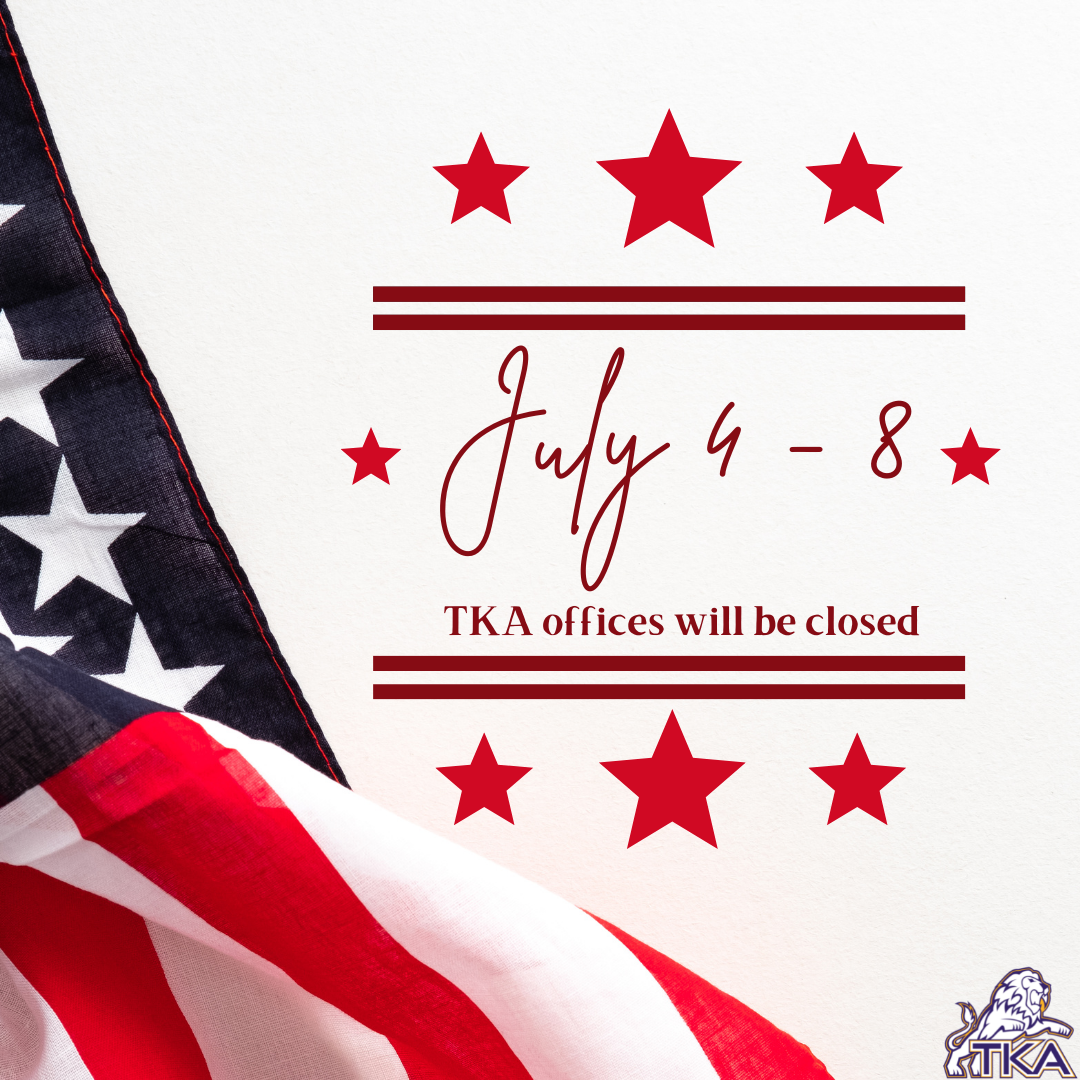# July 4th - 8th

In observation of July 4, The King's Academy offices will be closed Monday, July 4, through Friday, July 8.

 table div table+table+table+table+table+table+table+table div table{width:100%;padding:0}table div table+table+table+table+table+table+table+table div table img{width:96.23%;padding:0;float:none}table div table+table+table+table+table+table+table+table div table td{width:100%;padding:0 1.88% 18px}/* styles */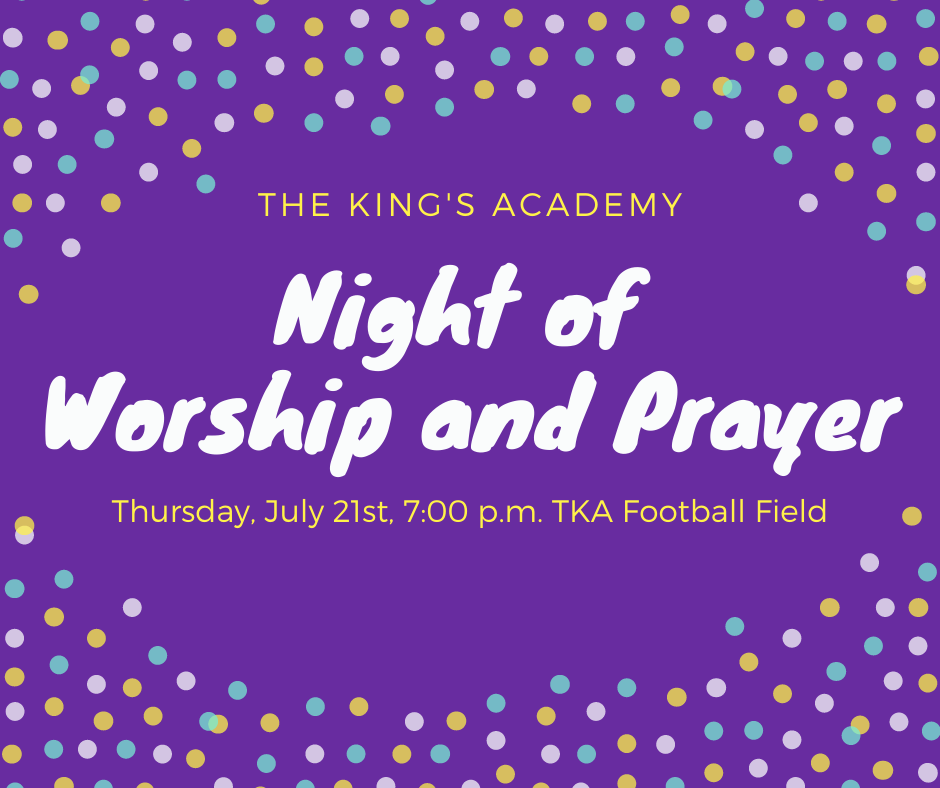# Night of Worship and Prayer

## Thursday, July 21

Mark your calendars and plan to join us on Thursday, July 21st at 7:00 p.m. on TKA's Huskey Football Field. We will take some time to pray for the upcoming school year and participate in worship as we ask for the Lord's blessing. We are thankful for the members of local churches who are gathering with us in lifting up TKA to the Lord. All members of the TKA family are invited to join us as we gather together.

 table div table+table+table+table+table+table+table+table+table+table div table{width:100%;padding:0}table div table+table+table+table+table+table+table+table+table+table div table img{width:96.23%;padding:0;float:none}table div table+table+table+table+table+table+table+table+table+table div table td{width:100%;padding:0 1.88% 18px}/* styles */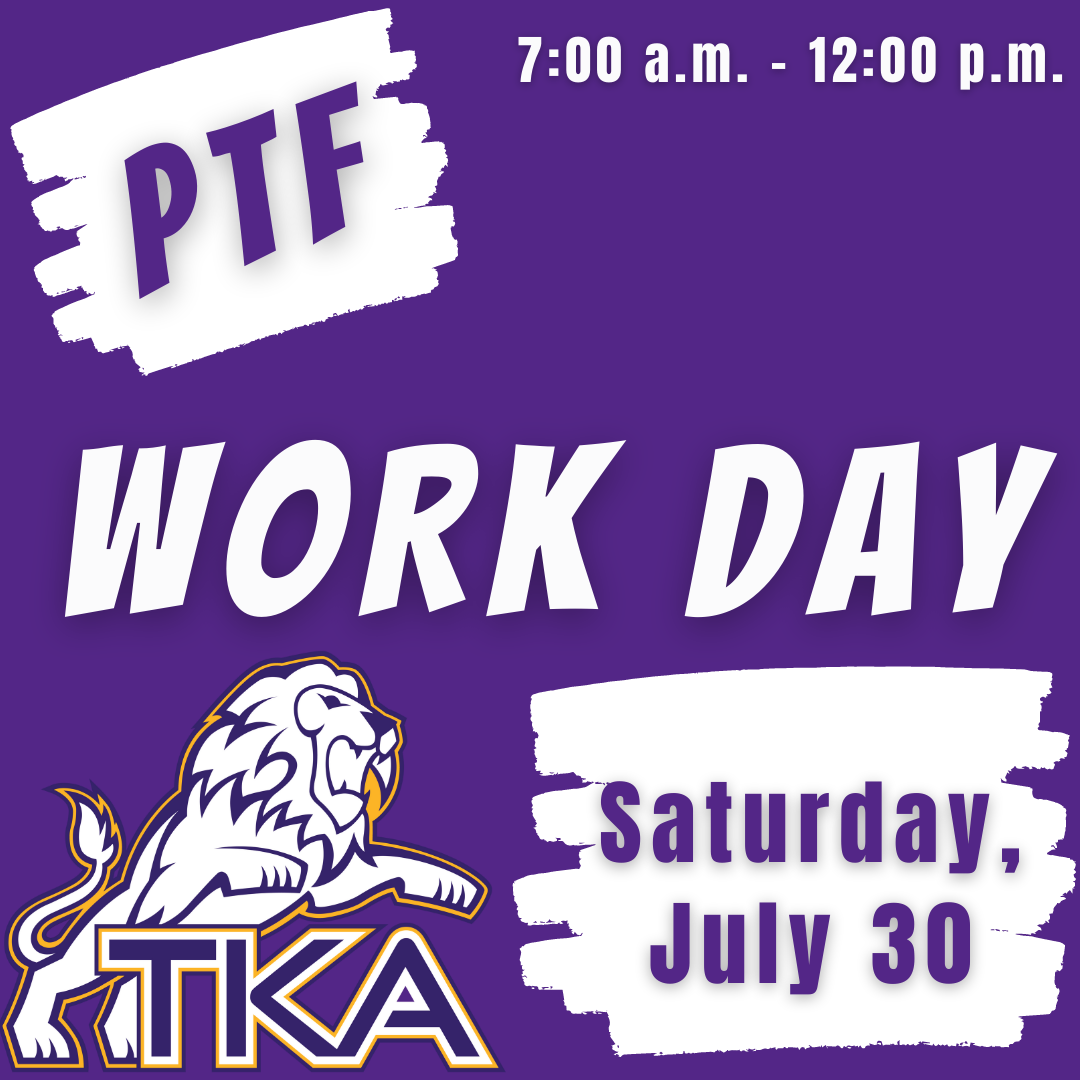# PTF Work Day

## Saturday, July 30

Join us on campus, Saturday, July 30, from 7:00 a.m. to 12:00 p.m. for a work day to help get the TKA campus ready for school to start!

 table div table+table+table+table+table+table+table+table+table+table+table+table div table{width:100%;padding:0}table div table+table+table+table+table+table+table+table+table+table+table+table div table img{width:96.23%;padding:0;float:none}table div table+table+table+table+table+table+table+table+table+table+table+table div table td{width:100%;padding:0 1.88% 18px}/* styles */# 2022 Summer Camps

We are excited to offer a variety of camps for our TKA Lions this summer! Each camp will be listed in the link below with links to register for each event. We are looking forward to seeing our Lions on campus at these fun camps!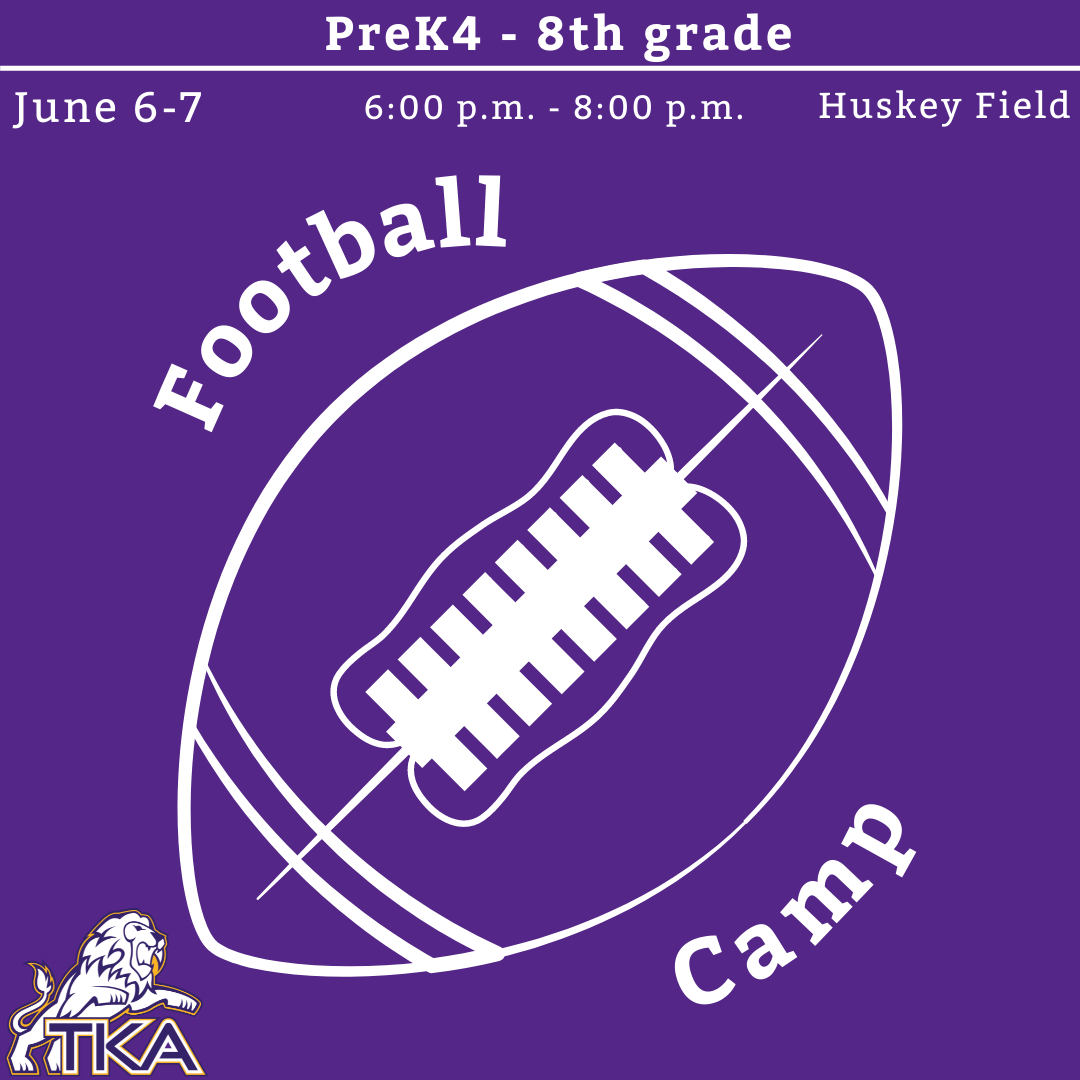# June 6-7: Youth Football Camp

Cost: \$20
Time: 6:00p.m. - 8:00p.m.
Location: Huskey Field

Athletes will be taught fundamental football skills through this camp.

 table div table+table+table+table+table+table+table+table+table+table+table+table+table+table+table+table div table{width:100%;padding:0}table div table+table+table+table+table+table+table+table+table+table+table+table+table+table+table+table div table img{width:96.23%;padding:0;float:none}table div table+table+table+table+table+table+table+table+table+table+table+table+table+table+table+table div table td{width:100%;padding:0 1.88% 18px}/* styles */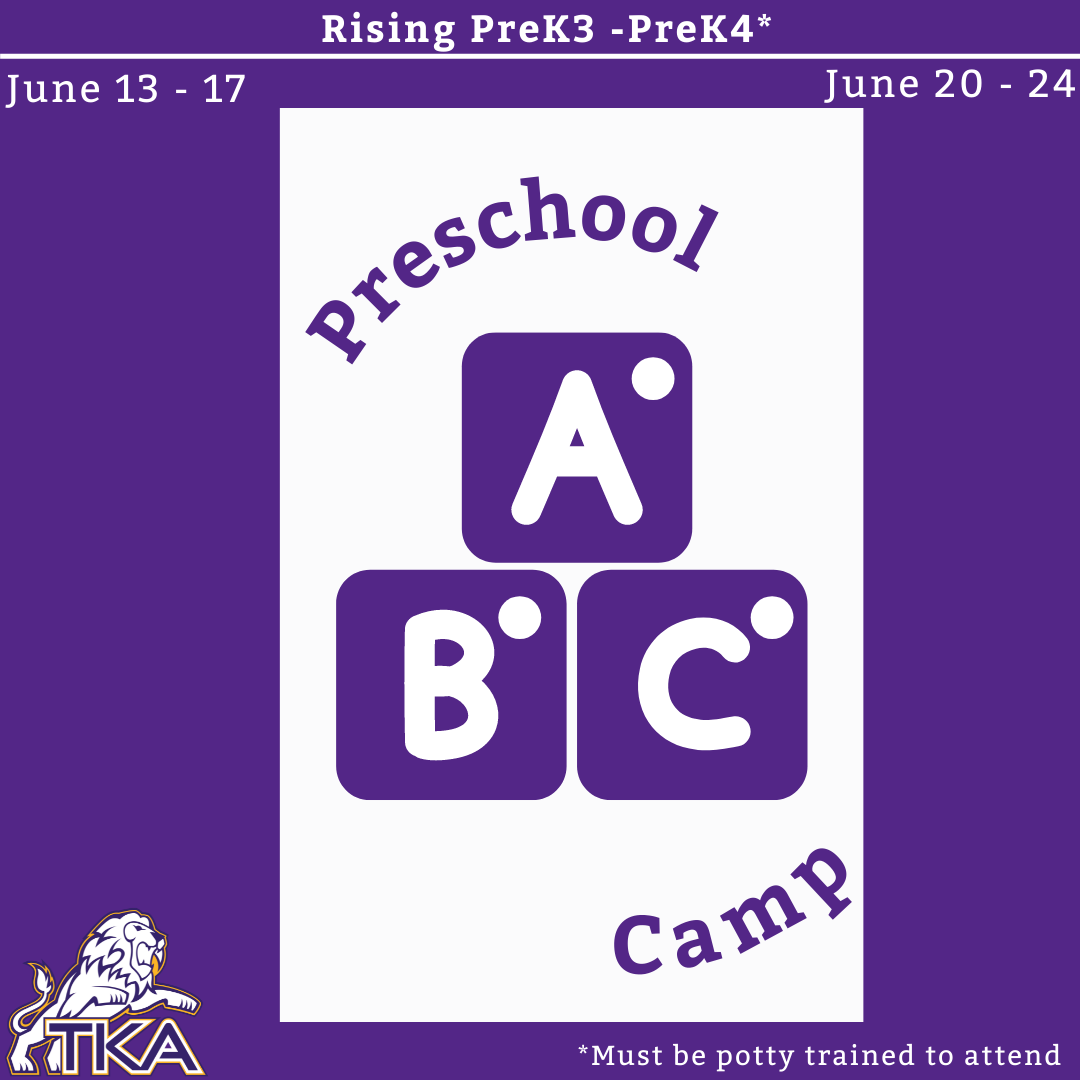# PreK Camp

## Rising PreK3 - PreK4 students*

*Attending preschoolers must be potty trained

Dates: June 13-17 & June 20-24
Cost: \$150
Time: 8:00 a.m. - 12:00 p.m.
Location: Ogle

Students will be learning through play and STEM activities. Throughout the week, students will be taught: letters, colors, crafts, and numbers. Please pack a snack each day for your child.

 table div table+table+table+table+table+table+table+table+table+table+table+table+table+table+table+table+table+table+table div table{width:100%;padding:0}table div table+table+table+table+table+table+table+table+table+table+table+table+table+table+table+table+table+table+table div table img{width:96.23%;padding:0;float:none}table div table+table+table+table+table+table+table+table+table+table+table+table+table+table+table+table+table+table+table div table td{width:100%;padding:0 1.88% 18px}/* styles */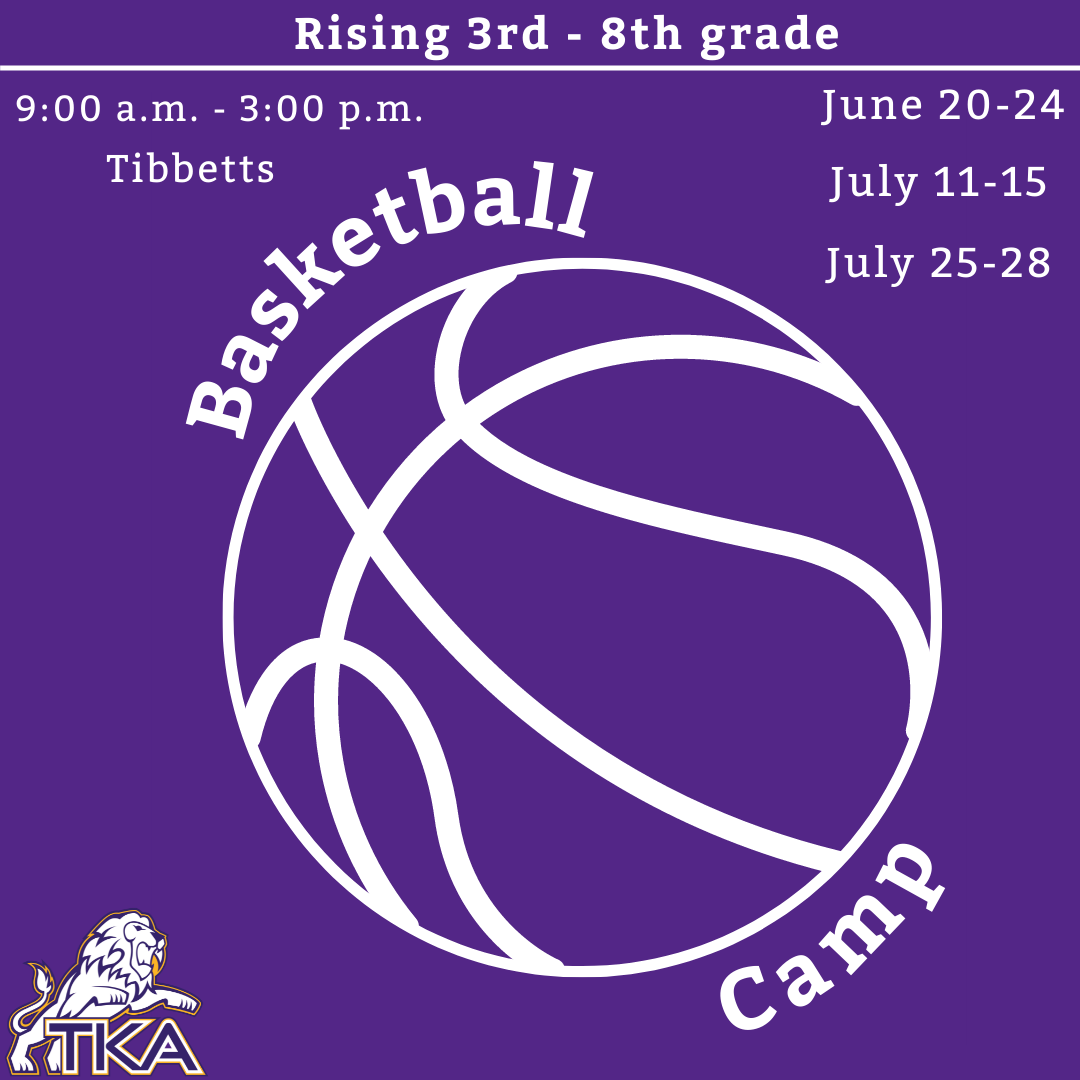## Rising 3rd - 8th students

Dates: June 20-24, July 11-15, July 25-28
Cost: \$120
Time: 9:00 a.m. - 3:00 p.m.
Location: Tibbetts

Athletes will have: individual instruction, Fundamental Skill stations, daily competitions, games (1v1, 3v3, and 5v5), and opportunities to purchase concessions.

 table div table+table+table+table+table+table+table+table+table+table+table+table+table+table+table+table+table+table+table+table+table+table div table{width:100%;padding:0}table div table+table+table+table+table+table+table+table+table+table+table+table+table+table+table+table+table+table+table+table+table+table div table img{width:96.23%;padding:0;float:none}table div table+table+table+table+table+table+table+table+table+table+table+table+table+table+table+table+table+table+table+table+table+table div table td{width:100%;padding:0 1.88% 18px}/* styles */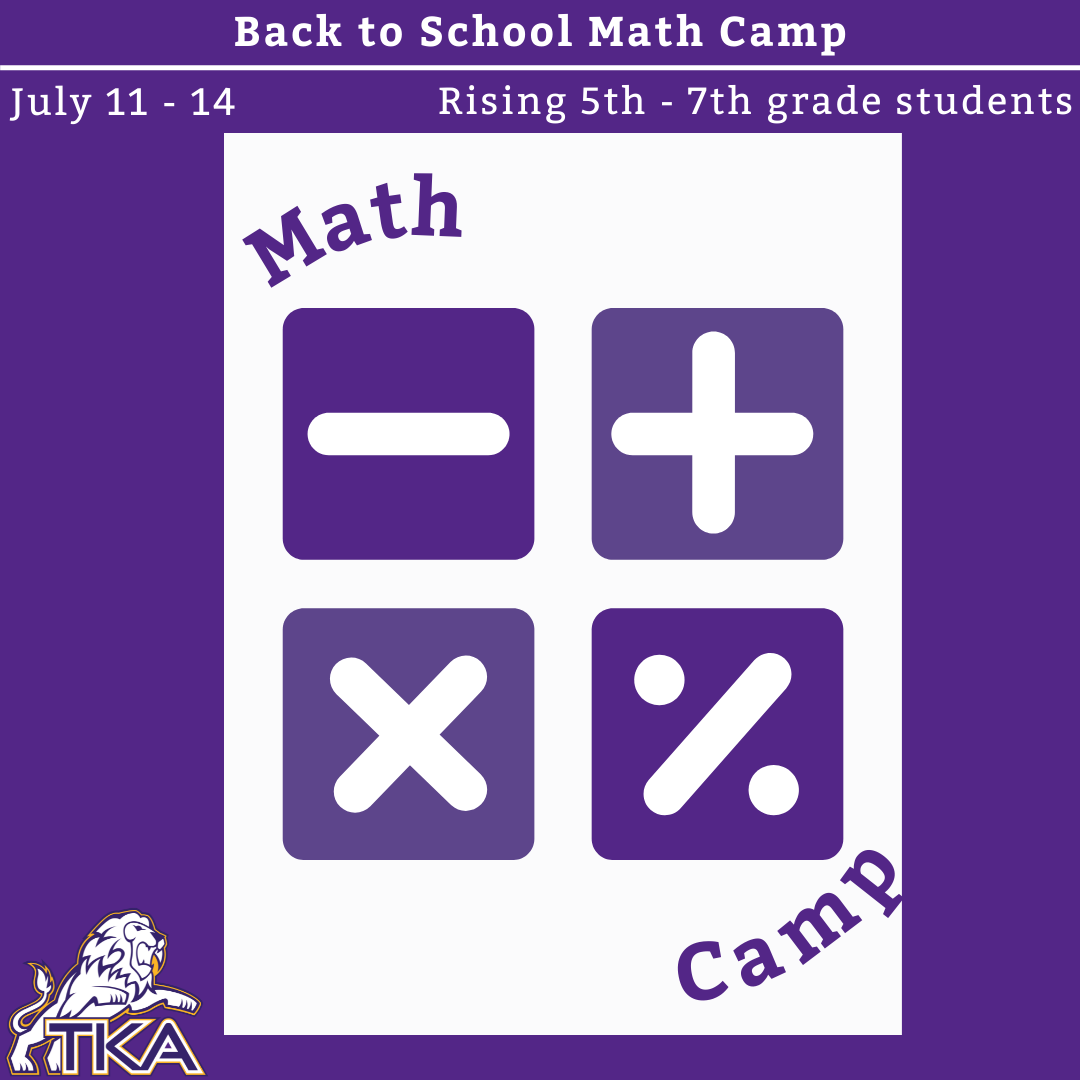# July 11-14: Back to School Math Camp

## Rising 5th - 7th students

Cost: \$160
Time: 9:00 a.m. - 12:00 p.m.
Location: Mrs. Sasscer’s room

A fun way to refresh and sharpen basic math skills! Please email or call Mrs. Sasscer or Mrs. Ailor with any questions.

 table div table+table+table+table+table+table+table+table+table+table+table+table+table+table+table+table+table+table+table+table+table+table+table+table+table div table{width:100%;padding:0}table div table+table+table+table+table+table+table+table+table+table+table+table+table+table+table+table+table+table+table+table+table+table+table+table+table div table img{width:96.23%;padding:0;float:none}table div table+table+table+table+table+table+table+table+table+table+table+table+table+table+table+table+table+table+table+table+table+table+table+table+table div table td{width:100%;padding:0 1.88% 18px}/* styles */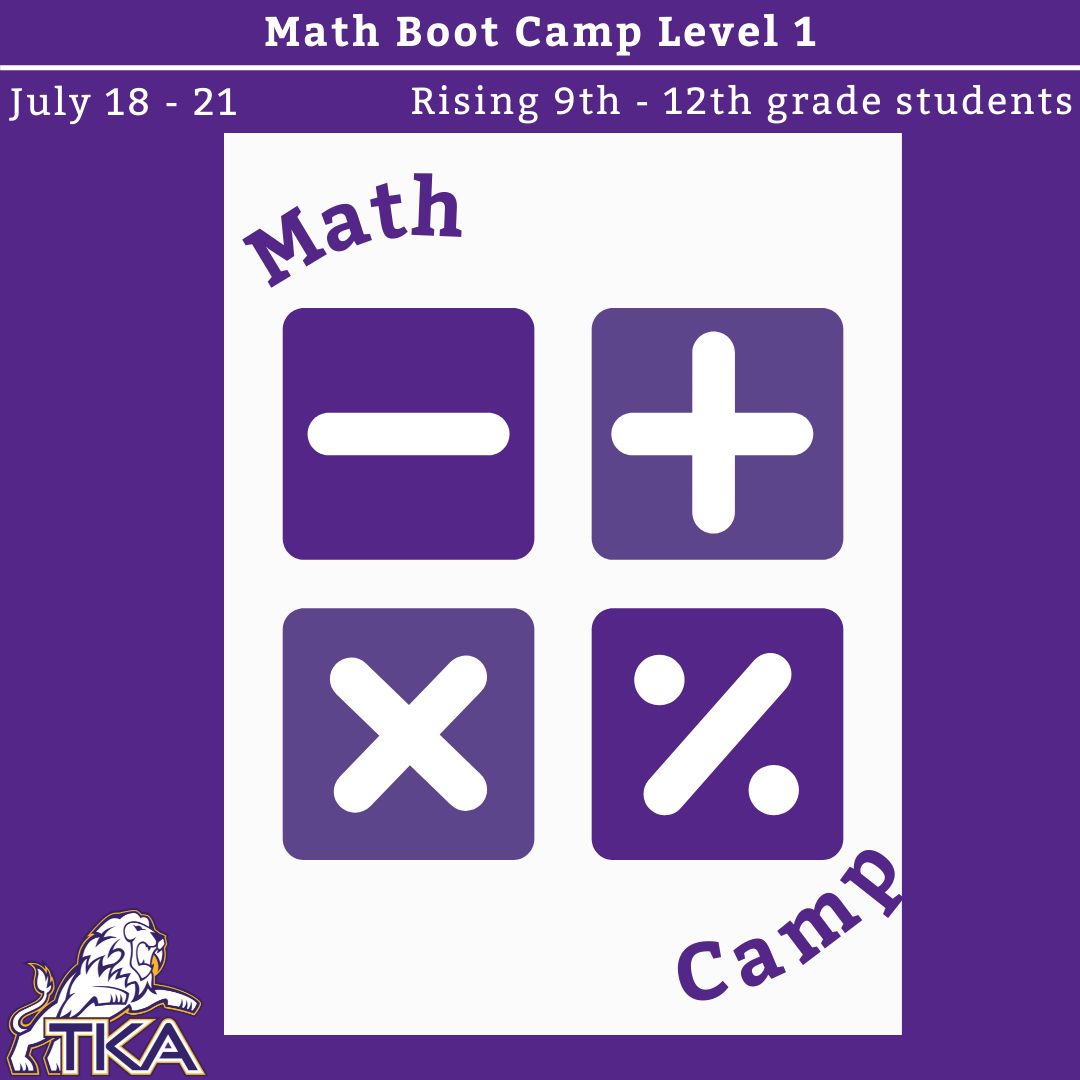# July 18-21: Math Boot Camp Level 1

## 8th - 11th grade students

Cost: \$200
Time: 9:00 a.m. - 12:00 p.m.
Location: Mrs. Moore’s room

Students will review: combining like terms, balancing equations, graphing linear equations, radicals, polynomial, quadratic, adding vs multiplying terms, exponent rules, distribution, fractions, complex functions, integers, functions and system of equations.

 table div table+table+table+table+table+table+table+table+table+table+table+table+table+table+table+table+table+table+table+table+table+table+table+table+table+table+table+table div table{width:100%;padding:0}table div table+table+table+table+table+table+table+table+table+table+table+table+table+table+table+table+table+table+table+table+table+table+table+table+table+table+table+table div table img{width:96.23%;padding:0;float:none}table div table+table+table+table+table+table+table+table+table+table+table+table+table+table+table+table+table+table+table+table+table+table+table+table+table+table+table+table div table td{width:100%;padding:0 1.88% 18px}/* styles */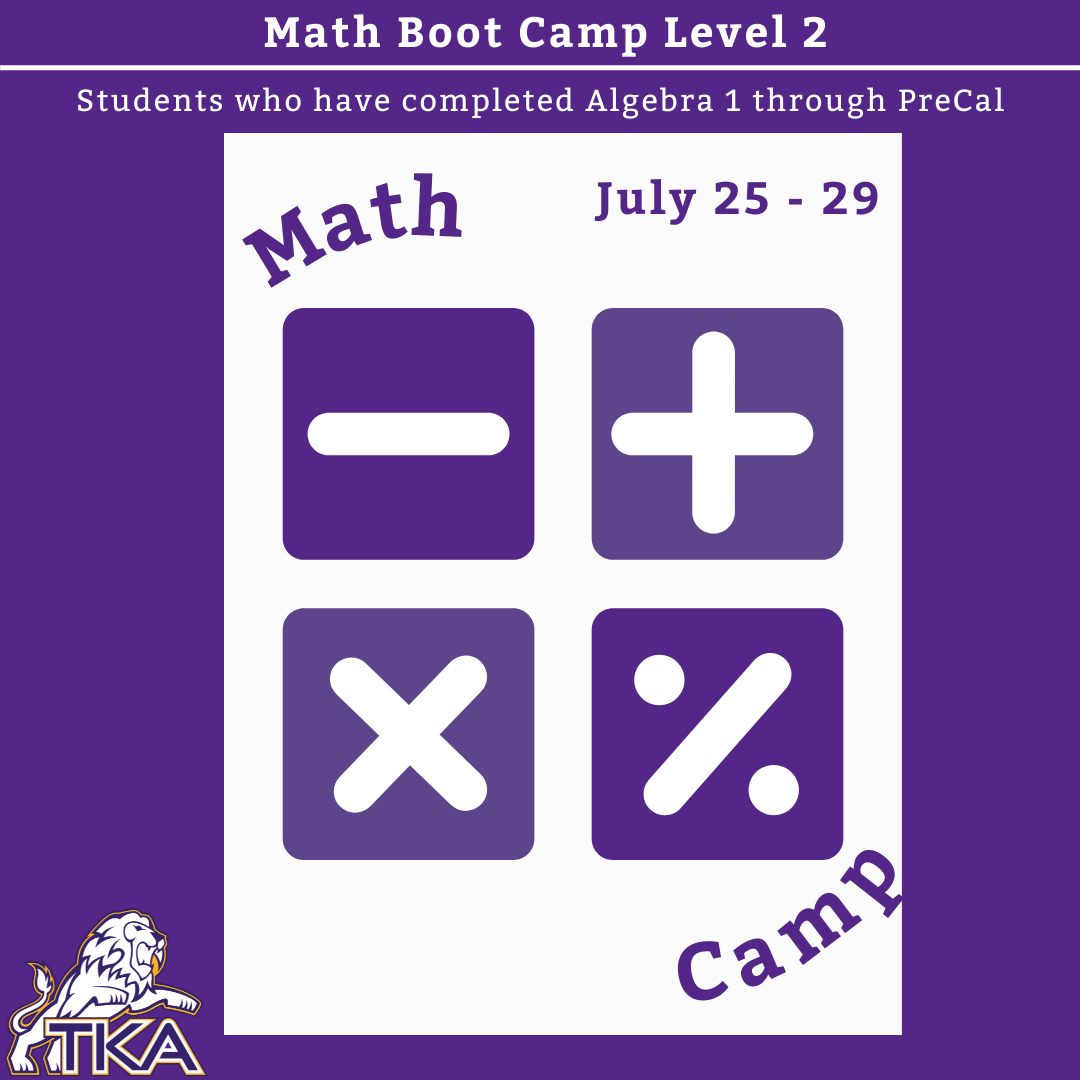# July 25-29: Math Boot Camp Level 2

## Students who have taken Algebra 1 through Pre- Cal

Cost: \$200
Time: 8:00 a.m. - 12:00 p.m.
Location: Mrs. Moore’s room

Students will review: fundamentals of Algebra and geometry, Trig ratios, unit circle, transformations related to linear, quadratic, exponential and absolute, domain, range, end behavior and complex radical expressions.

 table div table+table+table+table+table+table+table+table+table+table+table+table+table+table+table+table+table+table+table+table+table+table+table+table+table+table+table+table+table+table+table div table{width:100%;padding:0}table div table+table+table+table+table+table+table+table+table+table+table+table+table+table+table+table+table+table+table+table+table+table+table+table+table+table+table+table+table+table+table div table img{width:96.23%;padding:0;float:none}table div table+table+table+table+table+table+table+table+table+table+table+table+table+table+table+table+table+table+table+table+table+table+table+table+table+table+table+table+table+table+table div table td{width:100%;padding:0 1.88% 18px}/* styles */# Important information for the 2022-2023 School Year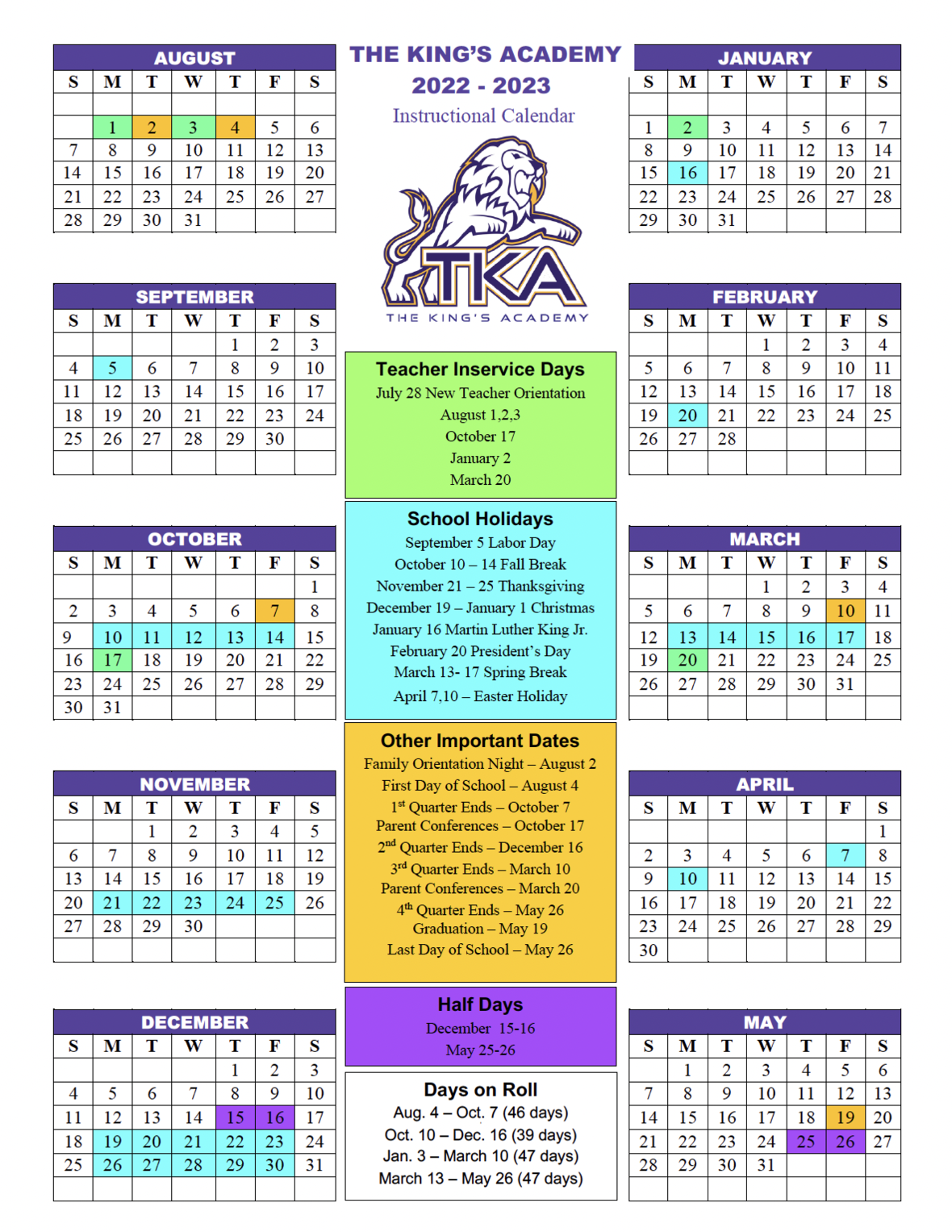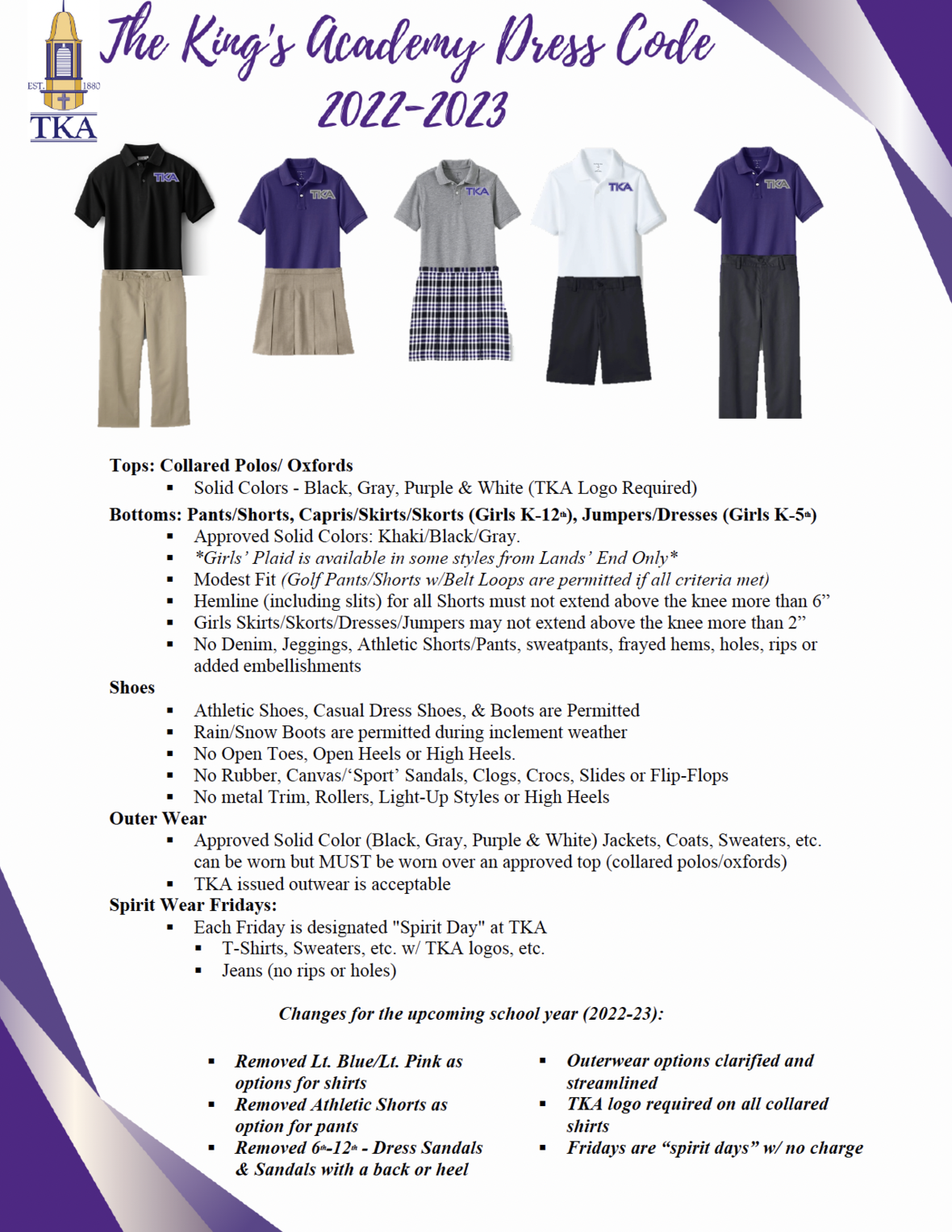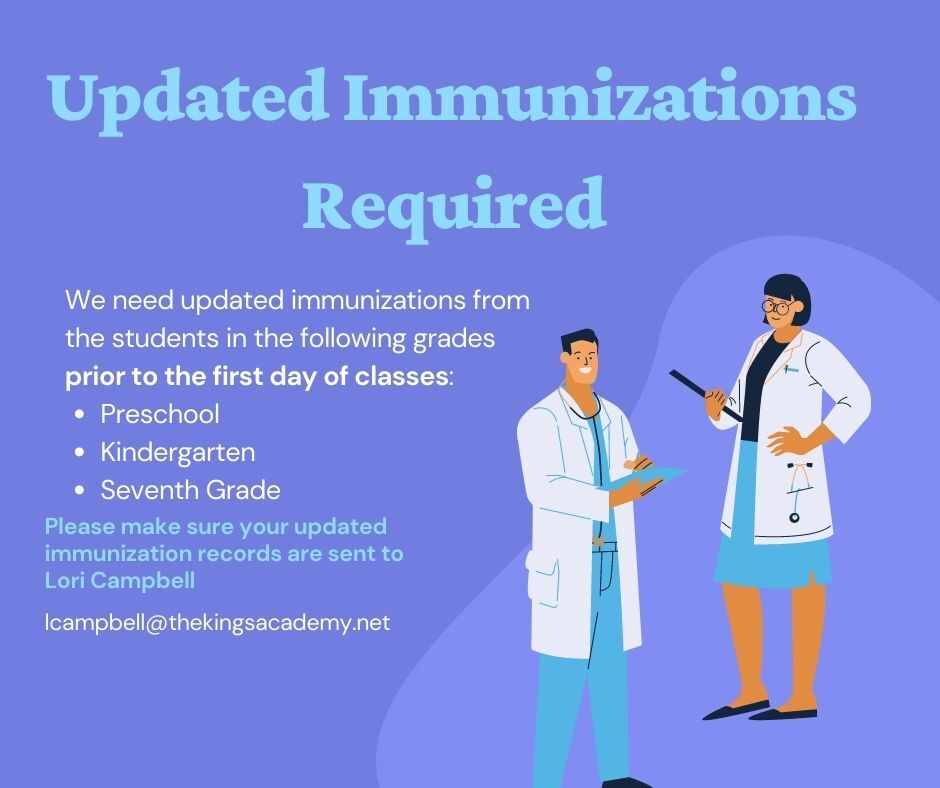table div table+table+table+table+table+table+table+table+table+table+table+table+table+table+table+table+table+table+table+table+table+table+table+table+table+table+table+table+table+table+table+table+table+table+table+table div table{width:100%;padding:0}table div table+table+table+table+table+table+table+table+table+table+table+table+table+table+table+table+table+table+table+table+table+table+table+table+table+table+table+table+table+table+table+table+table+table+table+table div table img{width:96.23%;padding:0;float:none}table div table+table+table+table+table+table+table+table+table+table+table+table+table+table+table+table+table+table+table+table+table+table+table+table+table+table+table+table+table+table+table+table+table+table+table+table div table td{width:100%;padding:0 1.88% 18px}/* styles */# Ways to help with the TKA Kenya Trip

Our team of TKA teachers will be serving the four hundred students of Empukani Primary (Kenya) in June by offering a Vacation Bible School along with teacher training. The focus of the VBS will be “I AM: The Promises of God.”

Many have asked how they could support the efforts of this trip through prayer and giving. The following items are needed and all donations are fully appreciated:

Snacks
· Vanilla wafers
· Twizzlers
· Goldfish
· Hard candy (not individually wrapped)

Crafts
· Embroidery floss (red, green, blue, yellow, and orange)
· Clear contact paper
· Colored sharpies
· White handkerchiefs (50 for \$20 on Amazon)
· Beads (black, red, green, white, yellow(gold), and blue)
· Pipe cleaners
· Crayons
· Markers
· Colored pencils
· Small sharpeners for colored pencils and crayons

We will have to pack these items and travel with them so we are looking for large sealed packages instead of individually wrapped packages. Ms. Shannon, Mrs. Smith, and Mrs. Q will be collecting these items in their classrooms; however, items can be dropped off in the High School or Elementary offices as well.

Any and all donations are truly appreciated!

Sincerely,

Kenya Mission Team

 table div table+table+table+table+table+table+table+table+table+table+table+table+table+table+table+table+table+table+table+table+table+table+table+table+table+table+table+table+table+table+table+table+table+table+table+table+table+table div table{width:100%;padding:0}table div table+table+table+table+table+table+table+table+table+table+table+table+table+table+table+table+table+table+table+table+table+table+table+table+table+table+table+table+table+table+table+table+table+table+table+table+table+table div table img{width:96.23%;padding:0;float:none}table div table+table+table+table+table+table+table+table+table+table+table+table+table+table+table+table+table+table+table+table+table+table+table+table+table+table+table+table+table+table+table+table+table+table+table+table+table+table div table td{width:100%;padding:0 1.88% 18px}/* styles */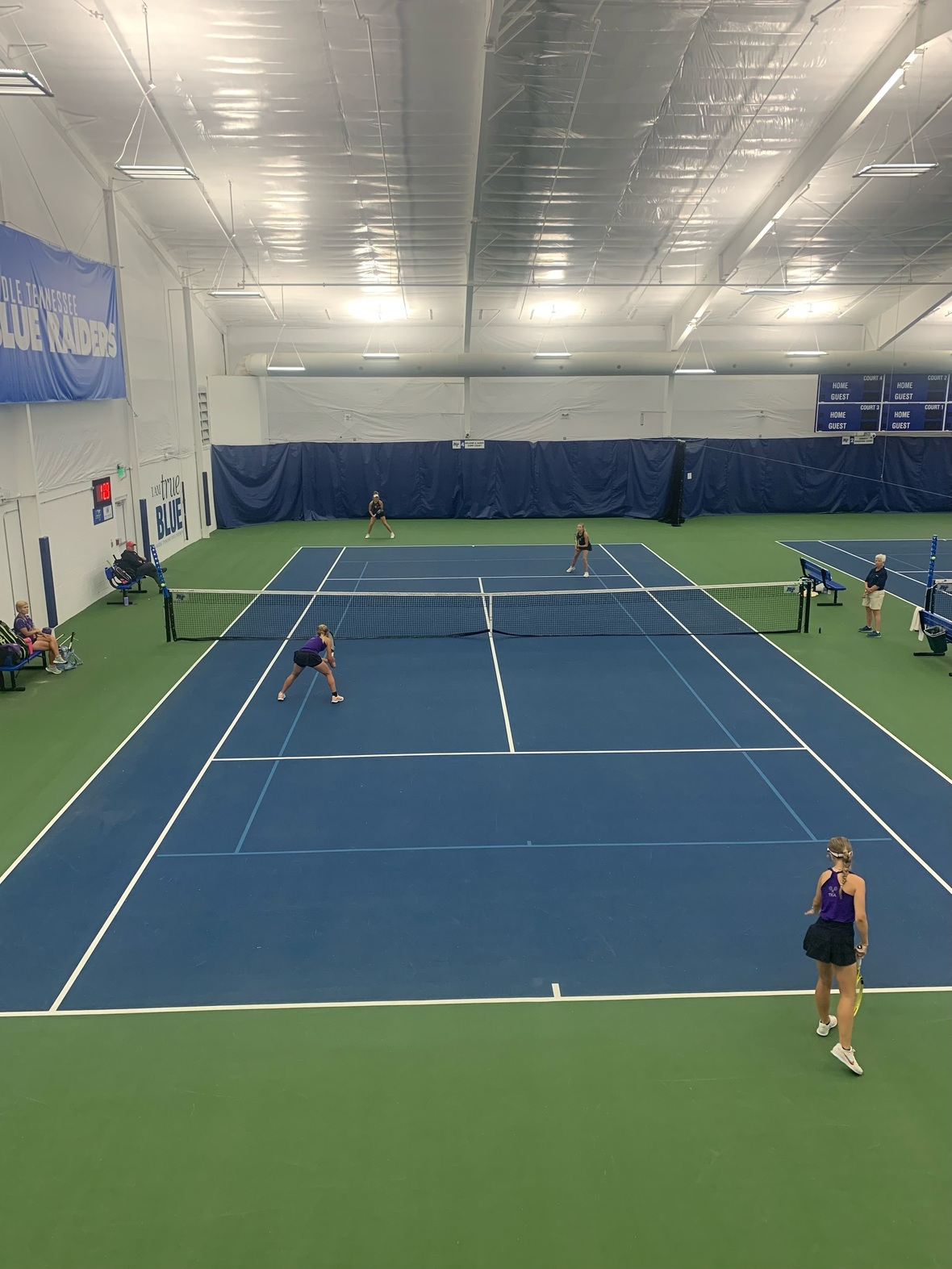# Tennis Highlight

Our Varsity girls, Kristen Dougherty and Katelyn Elizabeth Cook, represented TKA at the State semi-finals for doubles.

They played hard and represented TKA well. We are proud of both of them.

 table div table+table+table+table+table+table+table+table+table+table+table+table+table+table+table+table+table+table+table+table+table+table+table+table+table+table+table+table+table+table+table+table+table+table+table+table+table+table+table+table div table{width:100%;padding:0}table div table+table+table+table+table+table+table+table+table+table+table+table+table+table+table+table+table+table+table+table+table+table+table+table+table+table+table+table+table+table+table+table+table+table+table+table+table+table+table+table div table img{width:96.23%;padding:0;float:none}table div table+table+table+table+table+table+table+table+table+table+table+table+table+table+table+table+table+table+table+table+table+table+table+table+table+table+table+table+table+table+table+table+table+table+table+table+table+table+table+table div table td{width:100%;padding:0 1.88% 18px}/* styles */# Ferns for Sale

TKA Garden & Greenhouse is selling ferns! The small ferns are \$5 and large ferns are \$10.

 table div table+table+table+table+table+table+table+table+table+table+table+table+table+table+table+table+table+table+table+table+table+table+table+table+table+table+table+table+table+table+table+table+table+table+table+table+table+table+table+table+table+table div table{width:100%;padding:0}table div table+table+table+table+table+table+table+table+table+table+table+table+table+table+table+table+table+table+table+table+table+table+table+table+table+table+table+table+table+table+table+table+table+table+table+table+table+table+table+table+table+table div table img{width:96.23%;padding:0;float:none}table div table+table+table+table+table+table+table+table+table+table+table+table+table+table+table+table+table+table+table+table+table+table+table+table+table+table+table+table+table+table+table+table+table+table+table+table+table+table+table+table+table+table div table td{width:100%;padding:0 1.88% 18px}/* styles */TKA's football team will be selling 2022-2023 calendars. Save your advertising spot today!

Pricing for the Ads are as follows:

▪ \$750 Back Cover (Full Page)
▪ \$500 Full Page
▪ \$250 Half Page
▪ \$125 Quarter Page
▪ \$75 Side Page
 ▪ \$750 Back Cover (Full Page)
 ▪ \$500 Full Page
 ▪ \$250 Half Page
 ▪ \$125 Quarter Page
 ▪ \$75 Side Page

 table div table+table+table+table+table+table+table+table+table+table+table+table+table+table+table+table+table+table+table+table+table+table+table+table+table+table+table+table+table+table+table+table+table+table+table+table+table+table+table+table+table+table+table+table div table{width:100%;padding:0}table div table+table+table+table+table+table+table+table+table+table+table+table+table+table+table+table+table+table+table+table+table+table+table+table+table+table+table+table+table+table+table+table+table+table+table+table+table+table+table+table+table+table+table+table div table img{width:96.23%;padding:0;float:none}table div table+table+table+table+table+table+table+table+table+table+table+table+table+table+table+table+table+table+table+table+table+table+table+table+table+table+table+table+table+table+table+table+table+table+table+table+table+table+table+table+table+table+table+table div table td{width:100%;padding:0 1.88% 18px}/* styles */# Missing Keys?

A set of keys were found in the elementary parking lot. If you are missing keys, please contact Mrs. Gioioso in the elementary office at tgioioso@thekingsacademy.net.

 table div table+table+table+table+table+table+table+table+table+table+table+table+table+table+table+table+table+table+table+table+table+table+table+table+table+table+table+table+table+table+table+table+table+table+table+table+table+table+table+table+table+table+table+table+table+table div table{width:100%;padding:0}table div table+table+table+table+table+table+table+table+table+table+table+table+table+table+table+table+table+table+table+table+table+table+table+table+table+table+table+table+table+table+table+table+table+table+table+table+table+table+table+table+table+table+table+table+table+table div table img{width:96.23%;padding:0;float:none}table div table+table+table+table+table+table+table+table+table+table+table+table+table+table+table+table+table+table+table+table+table+table+table+table+table+table+table+table+table+table+table+table+table+table+table+table+table+table+table+table+table+table+table+table+table+table div table td{width:100%;padding:0 1.88% 18px}/* styles */# 27th Annual Golf Tournament

Join us at Egwani Farms on June 13, for our 27th Annual Golf Tournament to benefit TKA's football team!

Gather your company, friends or family and purchase one of the following sponsorships:
- Purple Sponsor includes a 4-person team and a hole sponsorship for \$500
- Silver Sponsor includes an individual player and a hole sponsorship for \$200
- Individual players can participate for \$100

 table div table+table+table+table+table+table+table+table+table+table+table+table+table+table+table+table+table+table+table+table+table+table+table+table+table+table+table+table+table+table+table+table+table+table+table+table+table+table+table+table+table+table+table+table+table+table+table+table div table{width:100%;padding:0}table div table+table+table+table+table+table+table+table+table+table+table+table+table+table+table+table+table+table+table+table+table+table+table+table+table+table+table+table+table+table+table+table+table+table+table+table+table+table+table+table+table+table+table+table+table+table+table+table div table img{width:96.23%;padding:0;float:none}table div table+table+table+table+table+table+table+table+table+table+table+table+table+table+table+table+table+table+table+table+table+table+table+table+table+table+table+table+table+table+table+table+table+table+table+table+table+table+table+table+table+table+table+table+table+table+table+table div table td{width:100%;padding:0 1.88% 18px}/* styles */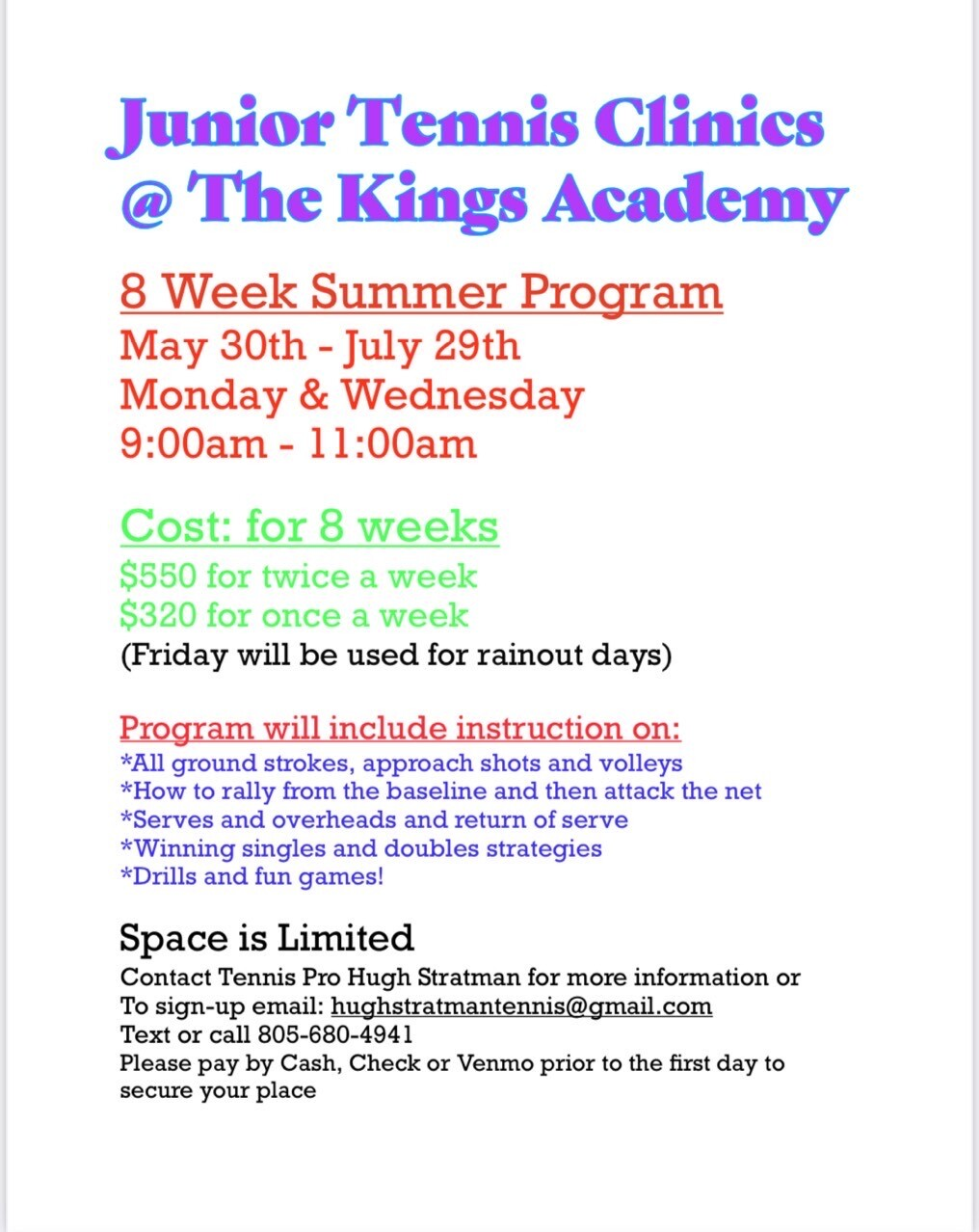# Jr. Tennis Clinics

## 8 week summer program

What: Tennis Pro Hugh Stratman will be offering an eight week tennis clinic.

Who: Middle and High school students

When: Mondays and Wednesdays from 9:00 a.m. to 11:00 a.m. starting May 30th through July 29th.

Where: TKA Tennis Courts

Cost (for 8 weeks): \$550 for twice a week
\$320 for once a week

How to sign up: Contact Hugh Stratman through text, phone call, or email at the contact information listed below:
Phone: 805-680-4941
Email: hughstratmantennis@gmail.com

 table div table+table+table+table+table+table+table+table+table+table+table+table+table+table+table+table+table+table+table+table+table+table+table+table+table+table+table+table+table+table+table+table+table+table+table+table+table+table+table+table+table+table+table+table+table+table+table+table+table+table div table{width:100%;padding:0}table div table+table+table+table+table+table+table+table+table+table+table+table+table+table+table+table+table+table+table+table+table+table+table+table+table+table+table+table+table+table+table+table+table+table+table+table+table+table+table+table+table+table+table+table+table+table+table+table+table+table div table img{width:96.23%;padding:0;float:none}table div table+table+table+table+table+table+table+table+table+table+table+table+table+table+table+table+table+table+table+table+table+table+table+table+table+table+table+table+table+table+table+table+table+table+table+table+table+table+table+table+table+table+table+table+table+table+table+table+table+table div table td{width:100%;padding:0 1.88% 18px}/* styles */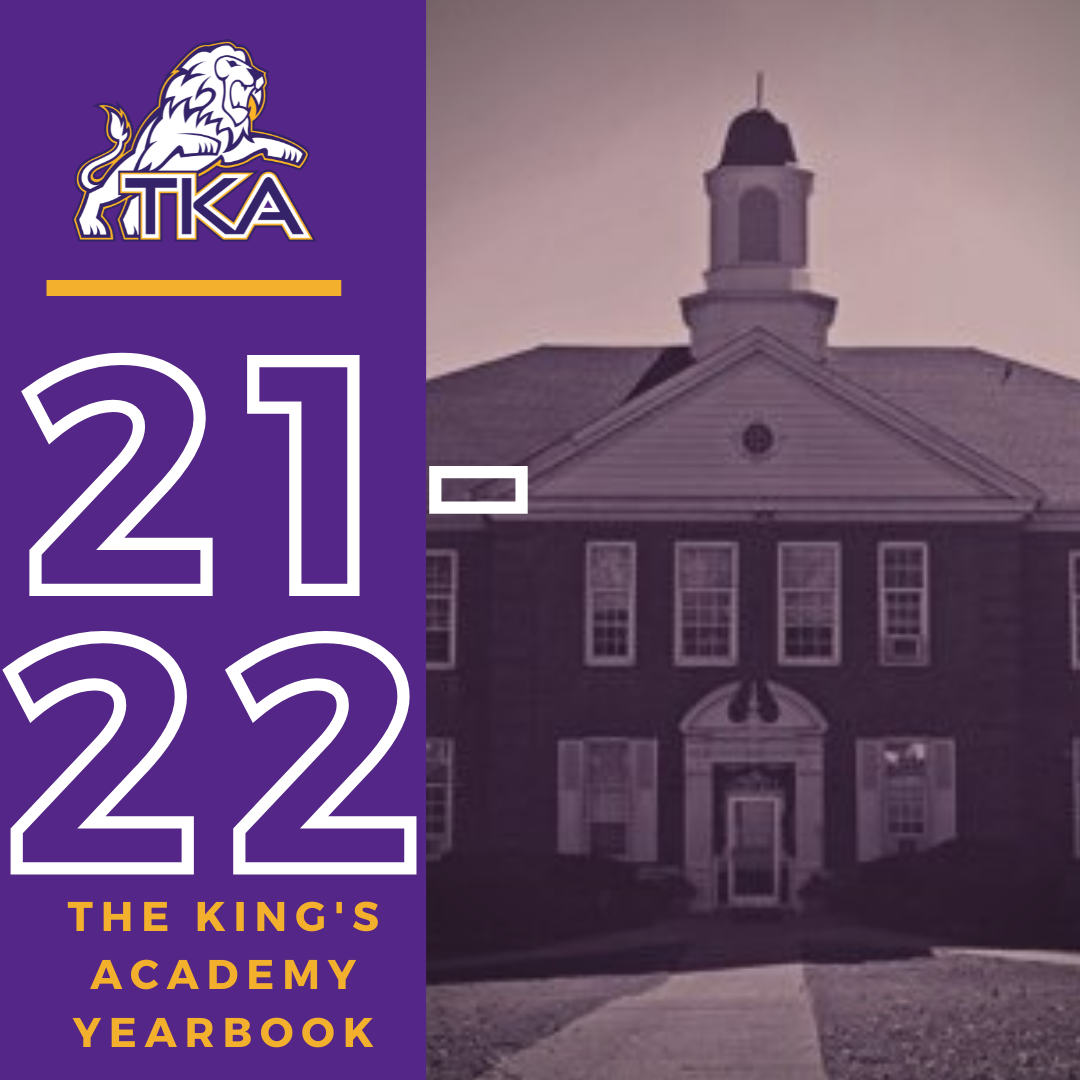# 2021-2022 Yearbook

The price for the 2021-2022 TKA yearbook is \$55. All grades, K3-12 will have the same yearbook. The slight price increase is because we are only going to have one larger all-school book.

All yearbook orders can be placed online--here is the link.Do you have a class of 2022 senior in your household?

Don't miss out on the opportunity to purchase a Senior Tribute for the 2021-2022 yearbook!

Senior Tributes are available for purchase at the bottom of the link listed here.table div table+table+table+table+table+table+table+table+table+table+table+table+table+table+table+table+table+table+table+table+table+table+table+table+table+table+table+table+table+table+table+table+table+table+table+table+table+table+table+table+table+table+table+table+table+table+table+table+table+table+table+table+table+table div table{width:100%;padding:0}table div table+table+table+table+table+table+table+table+table+table+table+table+table+table+table+table+table+table+table+table+table+table+table+table+table+table+table+table+table+table+table+table+table+table+table+table+table+table+table+table+table+table+table+table+table+table+table+table+table+table+table+table+table+table div table img{width:96.23%;padding:0;float:none}table div table+table+table+table+table+table+table+table+table+table+table+table+table+table+table+table+table+table+table+table+table+table+table+table+table+table+table+table+table+table+table+table+table+table+table+table+table+table+table+table+table+table+table+table+table+table+table+table+table+table+table+table+table+table div table td{width:100%;padding:0 1.88% 18px}/* styles */table div table+table+table+table+table+table+table+table+table+table+table+table+table+table+table+table+table+table+table+table+table+table+table+table+table+table+table+table+table+table+table+table+table+table+table+table+table+table+table+table+table+table+table+table+table+table+table+table+table+table+table+table+table+table+table+table+table div table{width:100%;padding:0}table div table+table+table+table+table+table+table+table+table+table+table+table+table+table+table+table+table+table+table+table+table+table+table+table+table+table+table+table+table+table+table+table+table+table+table+table+table+table+table+table+table+table+table+table+table+table+table+table+table+table+table+table+table+table+table+table+table div table img{width:96.23%;padding:0;float:none}table div table+table+table+table+table+table+table+table+table+table+table+table+table+table+table+table+table+table+table+table+table+table+table+table+table+table+table+table+table+table+table+table+table+table+table+table+table+table+table+table+table+table+table+table+table+table+table+table+table+table+table+table+table+table+table+table+table div table td{width:100%;padding:0 1.88% 18px}/* styles */table div table+table+table+table+table+table+table+table+table+table+table+table+table+table+table+table+table+table+table+table+table+table+table+table+table+table+table+table+table+table+table+table+table+table+table+table+table+table+table+table+table+table+table+table+table+table+table+table+table+table+table+table+table+table+table+table+table+table+table div table{width:100%;padding:0}table div table+table+table+table+table+table+table+table+table+table+table+table+table+table+table+table+table+table+table+table+table+table+table+table+table+table+table+table+table+table+table+table+table+table+table+table+table+table+table+table+table+table+table+table+table+table+table+table+table+table+table+table+table+table+table+table+table+table+table div table img{width:96.23%;padding:0;float:none}table div table+table+table+table+table+table+table+table+table+table+table+table+table+table+table+table+table+table+table+table+table+table+table+table+table+table+table+table+table+table+table+table+table+table+table+table+table+table+table+table+table+table+table+table+table+table+table+table+table+table+table+table+table+table+table+table+table+table+table div table td{width:100%;padding:0 1.88% 18px}/* styles */